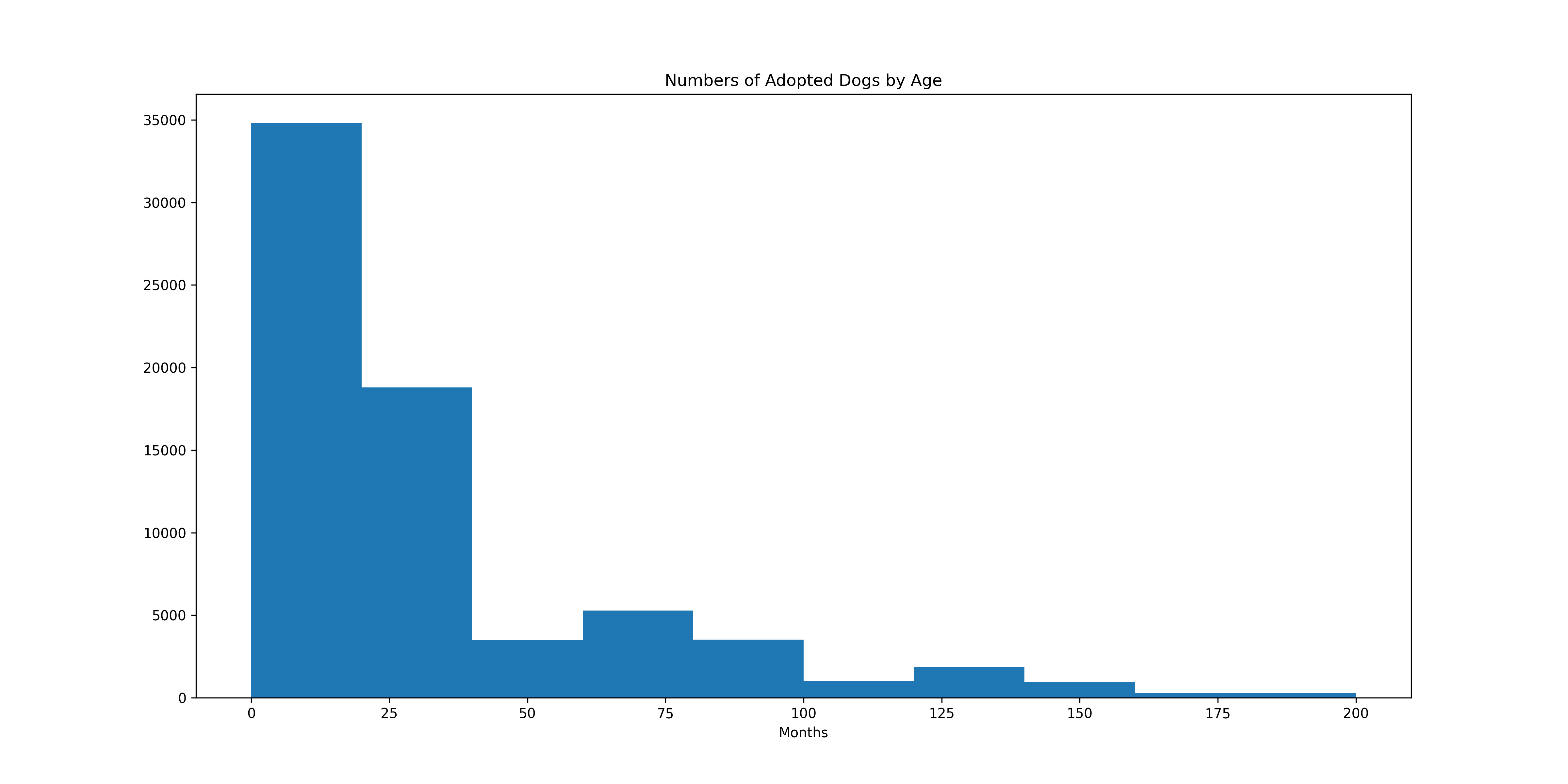# .hist()

The `.hist()` method in the matplotlib library is used to draw a histogram plot, showing the frequency of values within a given range.

## Syntax

``````matplotlib.pyplot.hist(x, bins, range, density, weights, cumulative, bottom, histtype, align, orientation, rwidth, log, color, label, stacked)
``````

The `x` argument is the only required parameter. It represents the values that will be plotted and can be of type float or array.

Other parameters are optional and can be used to customize plot elements such as the number of bins, color, and other relevant features.

`.hist()` takes the following arguments:

• `x`: Values to be plotted of type float or array.
• `bins`: Sets the number of bins, otherwise the number is autogenerated by default.
• `range`: The range of values to consider as a tuple in lieu of the entire range included in the data.
• `density`: Adds a probability density line (overlay) to the plot, default is `False`.
• `weights`: Accepts an array of values (must match the shape of `x`) that determines the relative contribution of each value in `x`.
• `cumulative`: Takes a boolean value, and if `True` computes a cumulative histogram where each bin gives counts of the relevant values within that bin in addition to all the values smaller than that bin.
• `bottom`: Sets the floor or the bottom of the bins, default is 0.
• `histtype`: Takes keywords (e.g. `bar`, `barstacked`, `step`, `stepfilled`) to modify the histogram type.
• `align`: Sets the alignment of the bins, default is `mid` (options include `left` and `right`).
• `orientation`: Sets the orientation of the histogram, default is `vertical`.
• `rwidth`: Sets the bar width as a fraction of the bin size.
• `log`: Changes the “y” values, the frequency of values, to a log scale.
• `color`: Sets the color of the lines/bars.
• `label`: Option to include names for multiple data sets.
• `stacked`: Boolean value that transforms the plot to be stacked, similar to a stacked bar graph.

## Example

The example below demonstrates the use of `.hist()` to plot a histogram figure.

```import pandas as pdimport matplotlib.pyplot as pltimport math
# Data is available at https://data.austintexas.gov/Health-and-Community-Services/Austin-Animal-Center-Intakes/wter-evkmdf = pd.read_csv('/content/Austin_Animal_Center_Intakes.csv')
# Function for standardizing pet age valuesdef get_months(x):  if 'week' in x:        return math.ceil(int(x.split(' ')) / 4 )  elif 'month' in x:      return int(x.split(' '))  elif 'year' in x:      return abs(int(x.split(' ')) * 12) # Some rows have negative values  else:      return 1 # If the entry is in days the function rounds up to 1 month
# Applying the function to create a new months columndf['months'] = df['Age upon Intake'].apply(get_months)
# Creating the plotplt.figure(figsize=(16,8))ax = plt.gca()
ax.hist(df.loc[df['Animal Type']=='Dog',['months']],range=(0,200))ax.set_title('Numbers of Adopted Dogs by Age')plt.xlabel('Months')plt.show()
```

Output: